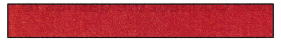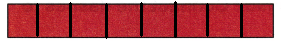# McGraw Hill Math Grade 2 Chapter 7 Lesson 4 Answer Key Dividing Rectangles into Squares

Practice questions available in McGraw Hill Math Grade 2 Answer Key PDF Chapter 7 Lesson 4 Dividing Rectangles into Squares will engage students and is a great way of informal assessment.

## McGraw-Hill Math Grade 2 Answer Key Chapter 7 Lesson 4 Dividing Rectangles into Squares

Count Parts

Count the squares. Write the number.

Question 1.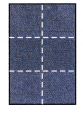6

Question 2.12

Explanation:
I counted by 1’s and there are 12 squares.

Question 3.
Circle the rectangle with 15 squares.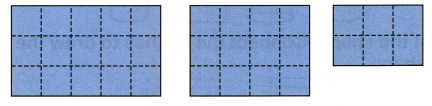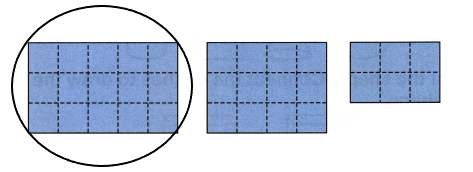Explanation:
I counted by 1’s and drew a circle around the rectangle with 15 squares
The first rectangle has 15 squares.

Question 4.
Draw lines on the rectangle. Make 8 squares.